# Texas Go Math Grade 6 Lesson 3.2 Answer Key Multiplying Mixed Numbers

Refer to our Texas Go Math Grade 6 Answer Key Pdf to score good marks in the exams. Test yourself by practicing the problems from Texas Go Math Grade 6 Lesson 3.2 Answer Key Multiplying Mixed Numbers.

## Texas Go Math Grade 6 Lesson 3.2 Answer Key Multiplying Mixed Numbers

Reflect

Question 1.
Make a Conjecture Will the product 1$$\frac{1}{2}$$ × $$\frac{1}{2}$$ be greater than or less than $$\frac{1}{2}$$? Explain.
We can solve this task using logic, or simply by doing the multiplication and seeing the result!

(1) Let’s think logically:
As we said before, when we multiply some number by a number that is greater than 1, our output will be greater than our original value.
Now, when we know which way to think, let’s check if 1$$\frac{1}{2}$$ is greater than 1.
1$$\frac{1}{2}$$ = 1 + $$\frac{1}{2}$$
Now it is clear, given mixed number is surely greater than 1.
Therefore, we can make our conclusion:
Product 1$$\frac{1}{2}$$ × $$\frac{1}{2}$$ will be greater than $$\frac{1}{2}$$.

(2) Using exact calculation to prove facts:
Let’s calculate the product of given expressions!
$$\frac{3}{2} \times \frac{1}{2}=\frac{3 \times 1}{2 \times 2}$$
= $$\frac{3}{4}$$
All that is left to do, is to compare our result with the original value!
Number 3 is greater than 2.
So we can conclude that:
Number $$\frac{3}{4}$$ is greater than $$\frac{1}{2}$$.

Exam stuff:
1$$\frac{1}{2}$$ = 1 + $$\frac{1}{2}$$
= $$\frac{2}{2}+\frac{1}{2}$$
= $$\frac{3}{2}$$

$$\frac{1}{2}$$ = $$\frac{1 \times 2}{2 \times 2}$$
= $$\frac{2}{4}$$

Multiply. Write each product in simplest form.

Question 2.
3$$\frac{1}{3}$$ × $$\frac{3}{4}$$ ________________
Process of multiplying fractions with mixed numbers
(1) Step:
Estimate the product Round the mixed number to the nearest whole number Find the nearest benchmark for the fraction.
3$$\frac{1}{3}$$ is close to 3, so multiply $$\frac{3}{4}$$ times 3.
3 × $$\frac{3}{4}$$ = $$\frac{3 \times 3}{4}$$
= $$\frac{9}{4}$$

(2) Step:
We transform mixed number into traction, and then multiply those tractions as in previous examples.Note that we will not do a transformation from mixed numbers into fractions as we can do it automatically now.
One more thing to add, the First step may not make sense at first, but it is crucial to find out whether our precise solution is correct!

Multiplying Two Mixed Numbers Lesson 3.2 Answer Key Question 3.
1$$\frac{4}{5}$$ × $$\frac{1}{2}$$ ________________
Process of multiplying fractions with mixed numbers
(1) Step:
Estimate the product Round the mixed number to the nearest whole number Find the nearest benchmark for the fraction.
1$$\frac{4}{5}$$ is close to 2, so multiply $$\frac{1}{2}$$ times 2.
$$\frac{1}{2}$$ × 2 = $$\frac{1 \times 2}{2}$$
= $$\frac{2}{2}$$ = 1

(2) Step:
We transform mixed numbers into traction and then multiply those tractions as in previous examples.Note that we will not do transformation from mixed number into fraction as we can do it automatically now.
One more thing to add, First step may not make sense at first, but it is crucial to find out whether our precise solution is correct!

Question 4.
$$\frac{5}{6}$$ × 2$$\frac{3}{4}$$ ________________
Process of multiplying fractions with mixed numbers
(1) Step:
Estimate the product Round the mixed number to the nearest whole number Find the nearest benchmark for the fraction.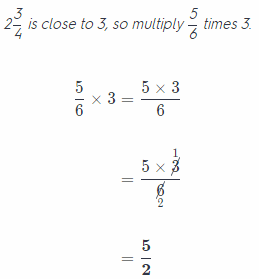(2) Step:
We transform mixed number into traction, and then multiply those tractions as in previous examples.Note that we will not do transformation from mixed number into fraction as we can do it automatically now.
One more thing to add, First step may not make sense at first, but it is crucial to find out whether our precise solution is correct!

Question 5.
$$\frac{3}{5}$$ × 2$$\frac{1}{5}$$ ________________
Process of multiplying fractions with mixed numbers
(1) Step:
Estimate the product Round the mixed number to the nearest whole number Find the nearest benchmark for the fraction.
2$$\frac{1}{5}$$ is close to 2, so multiply $$\frac{3}{5}$$ times 2.
$$\frac{3}{5}$$ × 2 = $$\frac{3 \times 2}{5}$$
= $$\frac{6}{5}$$

(2) Step:
We transform mixed number into traction, and then multiply those tractions as in previous examples.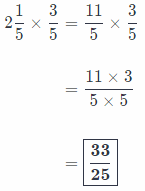Note that we will not do a transformation from mixed numbers into fractions as we can do it automatically now.
One more thing to add, the First step may not make sense at first, but it is crucial to find out whether our precise solution is correct!

Lesson 3.2 Go Math 6th Grade Go Math Question 6.
$$\frac{9}{10}$$ × 4$$\frac{1}{3}$$ ________________
Process of multiplying fractions with mixed numbers
(1) Step:
Estimate the product Round the mixed number to the nearest whole number Find the nearest benchmark for the fraction.
4$$\frac{1}{3}$$ is close to 4, so multiply $$\frac{9}{10}$$ times 4.(2) Step:
We transform mixed number into fraction, and then multiply those tractions as in previous examples.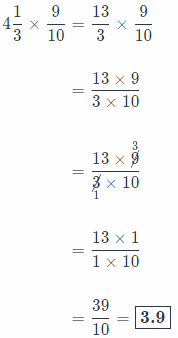Note that we will not do transformation from mixed number into fraction as we can do it automatically now.
One more thing to add, First step may not make sense at first, but it is crucial to find out whether our precise solution is correct!

Question 7.
5$$\frac{1}{6}$$ × $$\frac{1}{8}$$ ________________
Process of multiplying fractions with mixed numbers
(1) Step:
Estimate the product Round the mixed number to the nearest whole number Find the nearest benchmark for the fraction.
5$$\frac{1}{6}$$ is close to 5, so multiply $$\frac{1}{8}$$ times 5.
$$\frac{1}{8}$$ × 5 = $$\frac{1 \times 5}{8}$$
= $$\frac{5}{8}$$

(2) Step:
We transform mixed number into traction, and then multiply those tractions as in previous examples.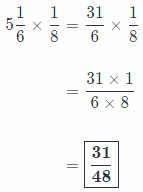Note that we will not do transformation from mixed number into fraction as we can do it automatically now.
One more thing to add, First step may not make sense at first, but it is crucial to find out whether our precise solution is correct!

Reflect

Question 8.
Analyze Relationships When you multiply two mixed numbers, will the product be less than or greater than the factors? Use an example to explain.
When we say that some number is mixed, it automatically gives us a clue.
Every mixed number is greater than 1, or at least its absolute value(we want to pay attention onLy on positive mixed numbers here).
So product of mixed numbers will be Greater than the factors.

Let’s show this on example: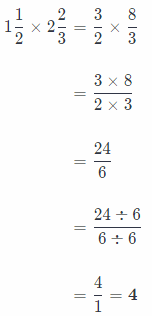From here, we can see that 4 is greater than both factors!

We would like to add one more side note here.
In previous tasks, we were rounding our mixed numbers to the nearest whole number
We skipped that part in our solutions, but we will write the explanation here.
So, how can we know whether we should round some mixed number to greater whole number or to less whole number?
Take a look at fraction, check if it is greater than or equal to a half, if it is, then round it to the greater whole number.
Otherwise, round it to the same whole number as it is given in mixed number.

Multiply. Write each product in simplest form.

Question 9.
2$$\frac{2}{3}$$ × 1$$\frac{1}{7}$$
First, we need to rewrite each mixed number as a fraction greater than 1. Then, we will multiply numerators and denominators. Final result we can write as a mixed number if it is greater than 1. So, applying all this, we have the following: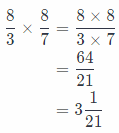the result is $$\frac{64}{21}$$ or 3$$\frac{1}{21}$$.

2$$\frac{3}{8}$$ × 1$$\frac{3}{5}$$
First, we need to rewrite each mixed number as a fraction greater than 1 and simplify before multiplying using the GCF and then rewrite fraction. Then, we will multiply numerators and denominators. Final result we can write as a mixed number if it is greater than 1. So, applying all this, we have the following: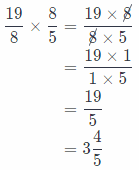So, the result is $$\frac{19}{5}$$ or 3$$\frac{4}{5}$$.

Question 11.
4$$\frac{1}{2}$$ × 3$$\frac{3}{7}$$
First, we need to rewrite each mixed number as a fraction greater than 1 and simplify before multiplying using the GCF and then rewrite fraction. Then, we will multiply numerators and denominators. Final result we can write as a mixed number if it is greater than 1. So, applying all this, we have the following:So, the result is $$\frac{108}{7}$$ or 15$$\frac{3}{7}$$.

Question 12.
5$$\frac{1}{4}$$ × 4$$\frac{2}{3}$$
First, we need to rewrite each mixed number as a fraction greater than 1 and simplify before multiplying using the GCF and then rewrite fraction. Then, we will multiply numerators and denominators. Final result we can write as a mixed number if it is greater than 1. So, applying all this, we have the following: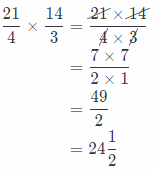So, the result is $$\frac{49}{2}$$ or 24$$\frac{1}{2}$$.

Question 1.
Mr. Martin’s yard is 1$$\frac{1}{3}$$ acres. He wants to plant grass on $$\frac{1}{6}$$ of his yard.

a. Draw a model to show how many acres will be covered by grass.On the following picture there is shown how many acres will be covered by grass.

b. How many acres will be covered by grass?
It will be covered $$\frac{2}{9}$$ acres by grass.

c. Write the multiplication shown by the model.
First, we need to rewrite each mixed number as a fraction greater than 1 and simplify before multiplying using the GCF and then rewrite fraction. Then, we will multiply numerators and denominators. Final result we can write as a mixed number if it is greater than 1. So, applying all this, we have the following:d. Will the mixed number that represents the original size of Mr. Martin’s yard increase or decrease when multiplied by $$\frac{1}{6}$$? Explain.
We can notice that Mr. Martin’s yard will decrease when multiply by $$\frac{1}{6}$$ because 0 < $$\frac{1}{6}$$ < 1 and when multiplying some number greater than 1 by number which is from interval (0, 1), product will decrease.

Multiply. Write each product in simplest form.

Question 2.
1$$\frac{1}{5}$$ × $$\frac{3}{5}$$
First, we need to rewrite each mixed number as a fraction greater than 1 and simplify before multiplying using the GCF and then rewrite the fraction. Then, we will multiply numerators and denominators. Final result we can write as a mixed number if it is greater than 1. So, applying all this, we have the following:
$$\frac{6}{5} \times \frac{3}{5}$$ = $$\frac{6 \times 3}{5 \times 5}$$
= $$\frac{18}{25}$$
So, the result is $$\frac{18}{25}$$

1$$\frac{3}{4}$$ × $$\frac{4}{7}$$
First, we need to rewrite each mixed number as a fraction greater than 1 and simplify before multiplying using the GCF and then rewrite the fraction. Then, we will multiply numerators and denominators. Final result we can write as a mixed number if it is greater than 1. So, applying all this, we have the following:So, the result is 1

Question 4.
1$$\frac{5}{6}$$ × $$\frac{2}{5}$$
First, we need to rewrite each mixed number as a fraction greater than 1 and simplify before multiplying using the GCF and then rewrite fraction. Then, we will multiply numerators and denominators. Final result we can write as a mixed number if it is greater than 1. So, applying all this, we have the following:So, the result is $$\frac{11}{15}$$

Question 5.
1$$\frac{7}{10}$$ × $$\frac{4}{5}$$
First, we need to rewrite each mixed number as a fraction greater than 1 and simplify before multiplying using the GCF and then rewrite fraction. Then, we will multiply numerators and denominators. Final result we can write as a mixed number if it is greater than 1. So, applying all this, we have the following:So, the result is $$\frac{34}{25}$$ = 1$$\frac{9}{25}$$

Question 6.
$$\frac{5}{9}$$ × 3$$\frac{9}{10}$$
First, we need to rewrite each mixed number as a fraction greater than 1 and simplify before multiplying using the GCF and then rewrite fraction. Then, we will multiply numerators and denominators. Final result we can write as a mixed number if it is greater than 1. So, applying all this, we have the following: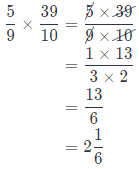So, the result is $$\frac{13}{6}$$ = 2$$\frac{1}{6}$$

Question 7.
$$\frac{7}{8}$$ × 3$$\frac{1}{3}$$
First, we need to rewrite each mixed number as a fraction greater than 1 and simplify before multiplying using the GCF and then rewrite fraction. Then, we will multiply numerators and denominators. Final result we can write as a mixed number if it is greater than 1. So, applying all this, we have the following:So, the result is $$\frac{35}{12}$$ = 2$$\frac{11}{12}$$

Question 8.
2$$\frac{1}{5}$$ × 2$$\frac{3}{5}$$
First, we need to rewrite each mixed number as a fraction greater than 1 and simplify before multiplying using the GCF and then rewrite fraction. Then, we will multiply numerators and denominators. Final result we can write as a mixed number if it is greater than 1. So, applying all this, we have the following: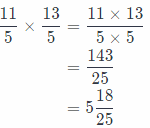So, the result is $$\frac{143}{25}$$ = 5$$\frac{18}{25}$$

Question 9.
4$$\frac{3}{4}$$ × 3$$\frac{4}{5}$$
First, we need to rewrite each mixed number as a fraction greater than 1 and simplify before multiplying using the GCF and then rewrite fraction. Then, we will multiply numerators and denominators. Final result we can write as a mixed number if it is greater than 1. So, applying all this, we have the following:So, the result is $$\frac{361}{20}$$ = 18$$\frac{1}{20}$$

Essential Question Check-In

Question 10.
How can you multiply two mixed numbers?
We can first rewrite the mixed number as a fraction grater than 1, if we are given mixed number Then simplify before multiplying using the GCF, if there are possibilities for this, after that rewrite fraction.

Finally, we multiply numerators and denominators and get the result which we can rewrite as a mixed number if it is grater than 1 and of course, if it is a fraction.

Rewrite mixed number as a fraction, simplify, multiply numerators and denominators.

Estimate. Then Solve.

Question 11.
Carly is making 3$$\frac{1}{2}$$ batches of biscuits. If one batch calls for 2$$\frac{1}{3}$$ cups of flour, how much flour will she need?
In order to find how much flour Carly will need, we have to multiply those mixed numbers.

First, we need to rewrite each mixed number as a fraction greater than 1 and simplify before multiplying using the GCF and then rewrite fraction. Then, we will multiply numerators and denominators. Final result we can write as a mixed number if it is greater than 1. So, applying all this, we have the following:So, conclusion is that Carly will need 12$$\frac{1}{4}$$ cups of flour.

Go Math Grade 6 Lesson 3.2 Multiplying Mixed Numbers Question 12.
Bashir collected 4$$\frac{1}{3}$$ baskets of peaches at an orchard. If each basket holds 21 peaches, how many peaches did he collect in all?
In order to find how much flour Carly will need, we have to multiply those mixed numbers.

First, we need to rewrite each mixed number as a fraction greater than 1 and simplify before multiplying using the GCF and then rewrite fraction. Then, we will multiply numerators and denominators. Final result we can write as a mixed number if it is greater than 1. So, applying all this, we have the following: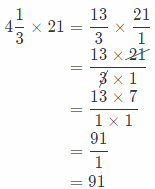Conclusion is that

Bashir collected 91 peach.

Question 13.
Jared used 1$$\frac{2}{5}$$ bags of soil for his garden. He is digging another garden that will need $$\frac{1}{5}$$ as much soil as the original. How much will he use total?
In order to calculate how much bags of soil Jared used total we have to multiply 1$$\frac{2}{5}$$ and $$\frac{1}{5}$$.
First we need to rewrite each mixed number as a fraction greater than 1 and simplify before multiplying using the GCF and then rewrite fraction. Then, we will multiply numerators and denominators. Final result we can write as a mixed number if it is greater than 1.

So, applying all this, we have the following:So, he used total $$\frac{7}{25}$$ bags of soil.

Question 14.
Critical Thinking is the product of two mixed numbers less than, between, or greater than the two factors? Explain.
If both fractions are greater than 1, or, if they are mixed numbers, then their product is greater than both fractions.
For example, 1$$\frac{1}{4}$$ = $$\frac{5}{4}$$ auch 1$$\frac{1}{5}$$ = $$\frac{6}{5}$$ are both greater than 1 and their product is following: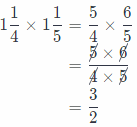And $$\frac{5}{4}$$ < $$\frac{6}{4}$$ = $$\frac{3}{2}$$, $$\frac{6}{5}$$ = $$\frac{12}{10}$$ < $$\frac{15}{10}$$ = $$\frac{3}{2}$$
Really, their product, $$\frac{3}{2}$$, is greater than both fractions.
If both fractions are less than 1, their product is less than both fractions.
For example, $$\frac{1}{4}$$ and $$\frac{3}{5}$$ are both less than 1 and their product is following: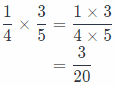And $$\frac{1}{4}=\frac{5}{20}>\frac{3}{20}, \frac{3}{5}=\frac{12}{20}>\frac{3}{20}$$

Really, their product, $$\frac{3}{20}$$, is less than both tractions.
And it one traction is greater than 1 or if it is mixed number and the other is less than 1, their product will be between those two tractions.
For example, $$\frac{1}{5}$$ is less than 1 and 1$$\frac{2}{5}$$ = $$\frac{7}{5}$$ is greater than 1, their product is following:
$$\frac{1}{5} \times \frac{7}{5}$$ = $$\frac{1}{5} \times \frac{7}{5}$$
= $$\frac{7}{25}$$
And $$\frac{5}{25}=\frac{1}{5}<\frac{7}{25}, \frac{30}{25}=\frac{6}{5}>\frac{7}{25}$$
Really, their product, $$\frac{7}{25}$$, is between those two fractions.

If both fractions are mixed numbers, fractions greater than 1, their product will be mixed number greater than both mixed numbers.
If both fractions are less than 1, their product will be less than both fractions.
If one fraction is less than 1 and the other is mixed number, greater than 1, their product wilL be between those two fractions.

Question 15.
There are approximately 402$$\frac{1}{4}$$ meters around a typical running track. Sandra has challenged herself to run 10 laps a day for 5 days. How many meters will Sandra run if she meets her challenge?
First we will calculate how many meters Sandra will run for a day.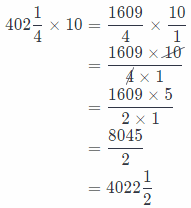Now, we will calculate how many meters Sandra wilt run for 5 days muLtiplying previous result by 5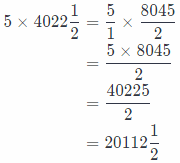So, Sandra will run 20112$$\frac{1}{2}$$ meters if she meets her challenge.

Question 16.
Ron wants to make a rectangular basketball court. What is the area of Ron’s court?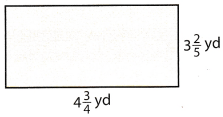The area of rectangular is product of length of its sides
Here, at this rectangular basketball court, one side is 4$$\frac{3}{4}$$ yd long and the other is 3$$\frac{2}{5}$$ yd long.
The area of this basketball court is the following:So, the area of Rou’s Court is 16$$\frac{3}{20}$$yd<sup>2</sup>

Question 17.
Each of 15 students will give a 1$$\frac{1}{2}$$-minute speech in English class.
a. How long will it take to give the speeches?
In order to calculate how long it will take to give the speeches, we have to multiply those two mixed numbers and get:So, it will take 22$$\frac{1}{2}$$ minutes to give the speeches.

b. If the teacher begins recording on a digital camera with an hour available, is there enough time to record everyone if she gives a 15-minute introduction at the beginning of class and every student takes a minute to get ready? Explain.
Because we have 15 students and every student takes a minute to get ready, on result from (a) we have to add this 15 × 1 = 15 minutes.
Also, we have to add 15 minutes more because that time is needed for introduction at the begining of class.
So, the whole ceremony with speeches wilL take: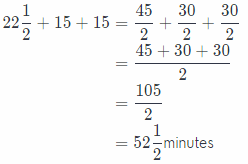Because 1 hour has 60 minutes and whole ceremony with speaches wiLl take 52$$\frac{1}{2}$$ minutes, which is less than 60 minutes, conclusion is that there will be enough time to record everyone on a digital camera.

c. How much time is left on the digital camera?
Now we will calculate how much time is left on the digital camera subtracting 52$$\frac{1}{2}$$, which is time needed for recording everyone, from 60 minutes, which is time for recording on digital camera.
So, we have the following: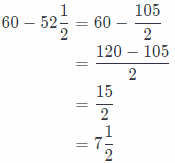So, there is 7$$\frac{1}{2}$$ minutes left on digital camera.

Question 18.
Communicate Mathematical Ideas How is multiplying a whole number by a mixed number the same as multiplying two mixed numbers?

H.O.T. Focus on Higher Order Thinking

Question 19.
Critique Reasoning To find the product 3$$\frac{3}{8}$$ x 4$$\frac{1}{9}$$, Tara rewrote 3$$\frac{3}{8}$$ as $$\frac{17}{8}$$ and 4$$\frac{1}{9}$$ as $$\frac{13}{2}$$. Then she multiplied the fractions to find the product $$\frac{221}{72}$$. What were her errors?
It is the same because whole number we need to rewrite as a fraction as welt as mixed numbers in order to multiply them.
We need to rewrite whole number as a fraction as well as mixed number.

Go Math Lesson 3.2 Grade 6 Multiply Mixed Fractions Question 20.
Represent Real-World Problems Ian is making his special barbecue sauce for a party. His recipe makes 3$$\frac{1}{2}$$ cups of barbecue sauce and uses 2$$\frac{1}{4}$$ tablespoons of soy sauce. He wants to increase his recipe to make five times as much barbecue sauce. He checks his refrigerator and finds that he has 8 tablespoons of soy sauce. Will he have enough soy sauce? Explain.
She did not rewrite mixed numbers properly as fractions, that was Tara’s mistake
The right way is the following:So, there product is actually $$\frac{111}{8}$$, or, 13$$\frac{7}{8}$$.

Question 21.
Analyze Relationships Is it possible to find the product of two mixed numbers by multiplying the whole number parts together, then multiplying the two fractional parts together, and finally adding the two products? Use an example to support your answer.
5 × 2$$\frac{1}{4}$$ = 5 × $$\frac{9}{4}$$
= $$\frac{5 \times 9}{1 \times 4}$$
= $$\frac{45}{4}$$